# Excel COUNTIF and COUNTIFS with OR conditions

The tutorial explains how to use Excel's COUNTIF and COUNTIFS functions to count cells with multiple OR as well as AND conditions.

As everyone knows, Excel COUNTIF function is designed to count cells based on just one criterion while COUNTIFS evaluates multiple criteria with AND logic. But what if your task requires OR logic - when several conditions are provided, any one can match to be included in the count?

There are a few possible solutions to this task, and this tutorial will cover them all in full detail. The examples imply that you have a sound knowledge of the syntax and general uses of both functions. If not, you may want to begin with revising the basics:

Excel COUNTIF function - counts cells with one criteria.

Excel COUNTIFS function - counts cells with multiple AND criteria.

Now that everyone is on the same page, let's dive in:

## Count cells with OR conditions in Excel

This section covers the simplest scenario - counting cells that meet any (at least one) of the specified conditions.

### Formula 1. COUNTIF + COUNTIF

The easiest way to count cells that have one value or another (Countif a or b) is to write a regular COUNTIF formula to count each item individually, and then add the results:

COUNTIF(range, criterion1) + COUNTIF(range, criterion2)

As an example, let's find out how many cells in column A contain either "apples" or "bananas":

`=COUNTIF(A:A, "apples") + COUNTIF(A:A, "bananas")`

In real-life worksheets, it is a good practice to operate on ranges rather than entire columns for the formula to work faster. To spare the trouble of updating your formula every time the conditions change, type the items of interest in predefined cells, say F1 and G1, and reference those cells. For example:

`=COUNTIF(A2:A10, F1) + COUNTIF(A2:A10, G1)`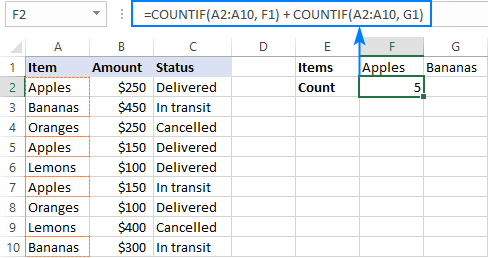This technique works fine for a couple of criteria, but adding three or more COUNTIF functions together would make the formula too cumbersome. In this case, you'd better stick with one of the following alternatives.

### Formula 2. COUNTIF with array constant

Here's a more compact version of the SUMIF with OR conditions formula in Excel:

SUM(COUNTIF(range, {criterion1, criterion2, criterion3, …}))

The formula is constructed in this way:

First, you package all the conditions in an array constant - individual items separated by commas and the array enclosed in curly braces like {"apples", "bananas', "lemons"}.

Then, you include the array constant in the criteria argument of a normal COUNTIF formula: COUNTIF(A2:A10, {"apples","bananas","lemons"})

Finally, warp the COUNTIF formula in the SUM function. It is necessary because COUNTIF will return 3 individual counts for "apples", "bananas" and "lemons", and you need to add those counts together.

Our complete formula goes as follows:

`=SUM(COUNTIF(A2:A10,{"apples","bananas","lemons"}))`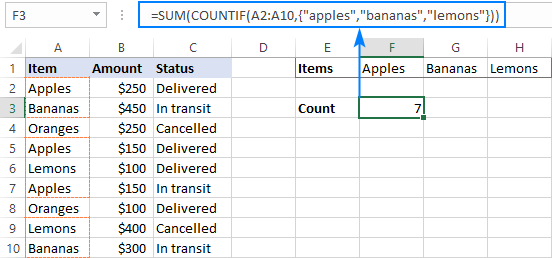If you'd rather supply your criteria as range references, you'll need to enter the formula with Ctrl + Shift + Enter to make it an array formula. For example:

`=SUM(COUNTIF(A2:A10,F1:H1))`

Please notice the curly braces in the screenshot below - it is the most evident indication of an array formula in Excel: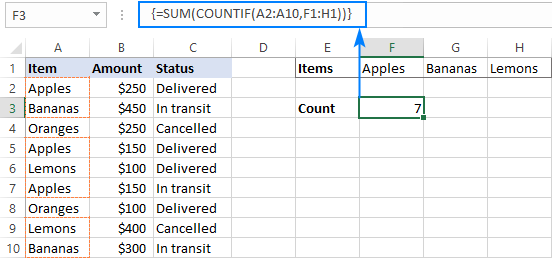### Formula 3. SUMPRODUCT

Another way to count cells with OR logic in Excel is to use the SUMPRODUCT function in this way:

SUMPRODUCT(1*(range ={criterion1, criterion2, criterion3, …}))

To better visualize the logic, this could also be written as:

SUMPRODUCT((range=criterion1) + (range=criterion2) + …)

The formula tests each cell in the range against each criterion and returns TRUE if the criterion is met, FALSE otherwise. As an intermediate result, you get a few arrays of TRUE and FALSE values (the number of arrays equals the number of your criteria). Then, the array elements in the same position are added together, i.e. the first elements in all the arrays, the second elements, and so on. The addition operation converts the logical values to numbers, so you end up with one array of 1's (one of the criteria matches) and 0's (none of the criteria matches). Because all the criteria are tested against the same cells, there is no way any other number could appear in the resulting array - only one initial array can have TRUE in a specific position, others will have FALSE. Finally, SUMPRODUCT adds up the elements of the resulting array, and you get the desired count.

The first formula works in a similar manner, with the difference that it returns one 2-dimentional array of TRUE and FALSE values, which you multiply by 1 to convert the logical values to 1 and 0, respectively.

Applied to our sample data set, the formulas take the following shape:

`=SUMPRODUCT(1*(A2:A10={"apples","bananas","lemons"}))`

Or

`=SUMPRODUCT((A2:A10="apples") + (A2:A10="bananas") + (A2:A10="lemons"))`

Replace the hardcoded array constant with a range reference, and you will get even a more elegant solution:

`=SUMPRODUCT(1*( A2:A10=F1:H1))`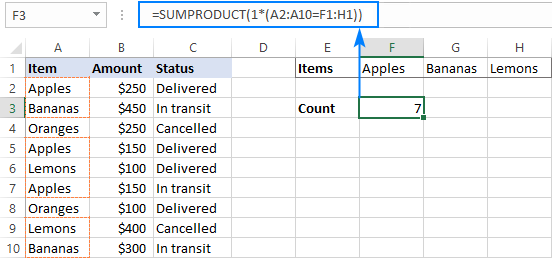Note. The SUMPRODUCT function is slower than COUNTIF, which is why this formula is best to be used on relatively small data sets.

## Count cells with OR as well as AND logic

When working with large data sets that have multi-level and cross-level relations between elements, chances are that you will need to count cells with OR and AND conditions at a time.

As an example, let's get a count of "apples", "bananas" and "lemons" that are "delivered". How do we do that? For starters, let's translate our conditions into Excel's language:

• Column A: "apples" or "bananas" or "lemons"
• Column C: "delivered"

Looking from another angle, we need to count rows with "apples and delivered" OR "bananas and delivered" OR "lemons and delivered". Put this way, the task boils down to counting cells with 3 OR conditions - exactly what we did in the previous section! The only difference is that you'll utilize COUNTIFS instead of COUNTIF to evaluate the AND criterion within each OR condition.

### Formula 1. COUNTIFS + COUNTIFS

It is the longest formula, which is the easiest to write :)

`=COUNTIFS(A2:A10, "apples", C2:C10, "delivered") + COUNTIFS(A2:A10, "bananas", C2:C10, "delivered")) + COUNTIFS(A2:A10, "lemons", C2:C10, "delivered"))`

The screenshot below shows the same formula with cells references:

`=COUNTIFS(A2:A10, K1, C2:C10, K2) + COUNTIFS(A2:A10, L1, C2:C10, K2) + COUNTIFS(A2:A10, M1,C2:C10, K2)`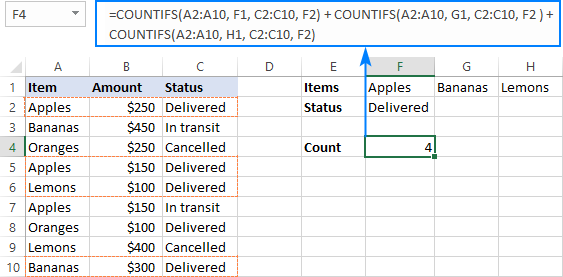### Formula 2. COUNTIFS with array constant

A more compact COUNTIFS formula with AND/OR logic can be created by packaging OR criteria in an array constant:

`=SUM(COUNTIFS(A2:A10, {"apples","bananas","lemons"}, C2:C10, "delivered"))`

When using a range reference for the criteria, you need an array formula, completed by pressing Ctrl + Shift + Enter:

`=SUM(COUNTIFS(A2:A10,F1:H1,C2:C10,F2))`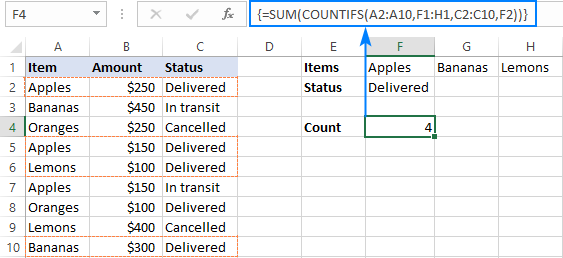Tip. If needed, you are free to use wildcards in the criteria of any formulas discussed above. For example, to count all sorts of bananas such as "green bananas" or "goldfinger bananas" you can use this formula:

`=SUM(COUNTIFS(A2:A10, {"apples","*bananas*","lemons"}, C2:C10, "delivered"))`

In a similar manner, you can build a formula to count cells based on other criteria types. For example, to get a count of "apples" or "bananas" or "lemons" that are "delivered" and the amount is greater than 200, add one more criteria range/criteria pair to COUNTIFS:

`=SUM(COUNTIFS(A2:A10, {"apples","*bananas*","lemons"}, C2:C10, "delivered", B2:B10, ">200"))`

Or, use this array formula (entered via Ctrl + Shift + Enter):

`=SUM(COUNTIFS(A2:A10,F1:H1,C2:C10,F2, B2:B10, ">"&F3))`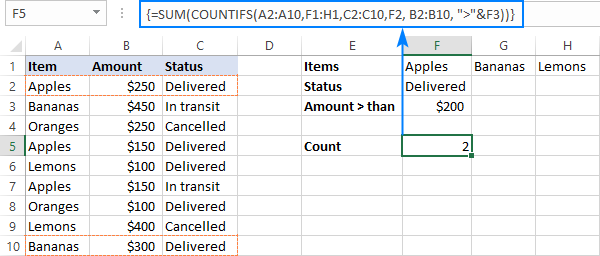## Count cells with multiple OR conditions

In the previous example, you have learned how to test one set of OR conditions. But what if you have two or more sets and you are looking to get a total of all possible OR relations?

Depending on how many conditions you need to handle, you can use either COUNTIFS with an array constant or SUMPRODUCT with ISNUMBER MATCH. The former is relatively easy to build, but it is limited to only 2 sets of OR conditions. The latter can evaluate any number of conditions (a reasonable number, of course, given Excel's limit to 255 arguments and 8192 characters to the total formula length), but it may take some effort to grasp the formula's logic.

### Count cells with 2 sets of OR conditions

When dealing with only two sets of OR criteria, just add one more array constant to the COUNTIFS formula discussed above.

For the formula to work, one minute but critical change is needed: use a horizontal array (elements separated by commas) for one criteria set and vertical array (elements separated by semicolons) for the other. This tells Excel to "pair" or "cross-calculate" the elements in the two arrays, and return a two-dimensional array of the results.

As an example, let's count "apples", "bananas" or "lemons" that are either "delivered" or "in transit":

`=SUM(COUNTIFS(A2:A10, {"apples", "bananas", "lemons"}, B2:B10, {"delivered"; "in transit"}))`

Please note the semicolon in the second array constant: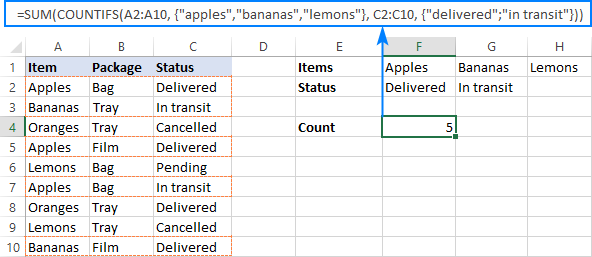Because Excel is a 2-dimentional program, it is not possible to construct a 3-dimentional or 4-dimentuional array, and therefore this formula only works for two sets of OR criteria. To count with more criteria, you will have to switch to a more complex SUMPRODUCT formula explained in the next example.

### Count cells with multiple sets of OR conditions

To count cells with more than two sets of OR criteria, use the SUMPRODUCT function together with ISNUMBER MATCH.

For example, let's get a count of "apples", "bananas" or "lemons" that are either "delivered" or "in transit" and are packaged in either "bag" or "tray":

```=SUMPRODUCT(ISNUMBER(MATCH(A2:A10,{"apples","bananas","lemons"},0))* ISNUMBER(MATCH(B2:B10,{"bag","tray"},0))* ISNUMBER(MATCH(C2:C10,{"delivered","in transit"},0))) ```

In the heart of the formula, the MATCH function checks the criteria by comparing each cell in the specified range with the corresponding array constant. If the match is found, it returns a relative position of the value if the array, N/A otherwise. ISNUMBER converts these values to TRUE and FALSE, which equate to 1 and 0, respectively. SUMPRODUCT takes it from there, and multiplies the arrays' elements. Because multiplying by zero gives zero, only the cells that have 1 in all the arrays survive and get summed.

Th screenshot below shows the result: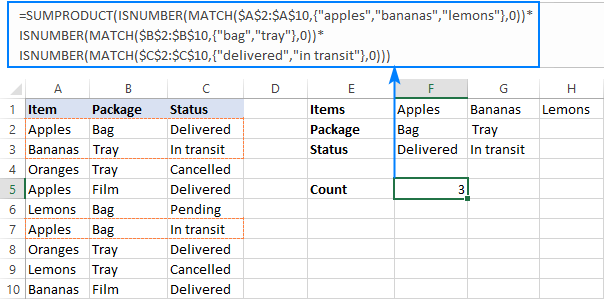This is how you use the COUNTIF and COUNTIFS functions in Excel to count cells with multiple AND as well as OR conditions. To have a closer look at the formulas discussed in this tutorial, you are welcome to download our sample Excel COUNTIF OR/AND workbook. I thank you for reading and hope to see you on our blog next week!

### 48 responses to "Excel COUNTIF and COUNTIFS with OR conditions"

1.Jonathan says:

Can you help me with a formula?

I have some student test scores and I'd like to create a formula that tells me how many students in each class got above the year average, and how many got below. But, there are some absent students, so I'd like to only count the scores above 0.

This is my current formula for below average scorers in class 1:

These formulas work, but the below average formula also includes scores for students who were absent and didn't take the test (so they include 0 scores and I'd like to change it).

My guess was that I should include COUNTIF(AD7:AD45,""&"0") somewhere in the formula, but every way I try isn't working. Do I need to do something different, like some sort of OR condition?

Jonathan

2.Jonathan says:

Can you help me with a formula?

I have some student test scores and I'd like to create a formula that tells me how many students in each class got above the year average, and how many got below. But, there are some absent students, so I'd like to only count the scores above 0.

This is my current formula for below average scorers in class 1:

These formulas work, but the below average formula also includes scores for students who were absent and didn't take the test (so they include 0 scores and I'd like to change it).

My guess was that I should include COUNTIF(AD7:AD45,""&"0") somewhere in the formula, but every way I try isn't working. Do I need to do something different, like some sort of OR condition?

Jonathan

•Doug says:

Jonathan:
You might be overthinking this.

3.Jan says:

Hi,

I may be under the wrong impression but, technically, all of your suggested solutions seem to work only as an AND fuction. That being said, your suggestions don't work well with my need of the real OR function in countif formula. Allow me to explain my problem.

I have a list of phrases in column A, position numbers in column B and another position numbers in column C. Now, I need to count all the phrases in column A that have the position <10 either in B or in C. The thing is that some phrases have both position numbers <10 and thus are being counted twice when using your solutions. That is why I need OR, not AND.

Surely, I could use filters to list and count those phrases "manually", but I need to make this process automated, as I am making basically the whole excel fill itself up based on my edits in one single sheet.

Thank you for your future suggestions.

•Svetlana Cheusheva (Ablebits.com Team) says:

Hi Jan,

A simple solution that comes to mind is to create a helper column (you can hide it or move to the end of the worksheet) and pull the smaller of the 2 values there: =MIN(B2:C2)

And then, you use COUNTIF on the helper column, e.g.=COUNTIF(D2:D100,"<"&10)

•Imran Masud says:

Thanks
many many love to you. This trick was really awesome.

4.Allen says:

Hi,

Column A contains various product names like Apple, Cherry, Banana, Grapes etc every product has more than 5 count in Column A
And Column B has only words like Bought and Sold for every other products

I want to create the formula to every product either of these

1. How many sold and bought for grapes, banana, apples etc
2. Which trade is greater is it Bought or Sold for every products.

Thanks,

Allen.

5.Brandon says:

I know this is a long shot however, I am currently having issues with creating a formula to read the following.
Name score1 score2 score3
smith 58 55 61

in the example above I need to create a formula that will count the number of cells w/ a score below 60. however, this issue I am having is I need the formula to only count this as a multiple failed event by one person and not read the formula as two cells <60, if that makes sense.
I have tried creating multiple formulas and cannot seem to get this to only count as one multiple failed event for the individual.

•Brandon says:

Some examples of formula I have been using are
=countif(E198:E262,"<60",G198:G262,"<60",I198:I262,"<60"). This formula only seems to count every score below 60. I need the formula to count multiple scores under 60 as 1 multiple failed event.

•Svetlana Cheusheva (Ablebits.com Team) says:

Hi Brandon,

And if a certain person has one or more scores under 60, what do you want to return? Simply 1, or something else?

6.James says:

Hi there!

I've been trying to formulate my sheet to count between numbers.

For example I have a host of different variables from 1.50 to around 100 and I wish to count them in sections, to explain further I want to count YES, NO VOID between 1.50 - 1.66 / 1.72 - 1.80 / 1.83 - 2.00 / 2.10 - 3.50 / 4.00 - 6.

I've tried to replicate these above formulas but most only count words and not numbers between ranges.

Can anyone provide any help?
Thank you

7.Tatyana says:

why not working countif(range,and()) ?

8.Valentyn says:

Hello.

A very good post and may have a solution for me as well. I've tried myself but could not get through.

So, I have 2 types of data in my table:
- some columns use only text and
- other columns use dates or text.

I need "COUNTIFS" with "OR" functionality for Date fields and WILDSCARDS (">=" or "<=" or "Blank"). For example, I need to use COUNTIFS with few OR and AND conditions in "Cell G1":
- ("Column B = Cell F1" OR "Column B = Blank"))

A bit messed explanation, but my difficulty is to add WILDCARDS ">=" or "<=" or "Blank" in your proposed formula.

9.Valentyn says:

did a repost since part of my post was not reflected above.

Hello.

A very good post and may have a solution for me as well. I've tried myself but could not get through.

So, I have 2 types of data in my table:
- some columns use only text and
- other columns use dates or text.

I need "COUNTIFS" with "OR" functionality for Date fields and WILDSCARDS (">=" or "<=" or "Blank"). For example, I need to use COUNTIFS with few OR and AND conditions in "Cell G1":
- ("Column B = Cell F1" OR "Column B = Blank"))

A bit messed explanation, but my difficulty is to add WILDCARDS ">=" or "<=" or "Blank" in your proposed formula.

10.Alex says:

Thanks so much for this tutorial, it was hugely helpful and very easy to follow!

I found that I didn't need SUM for the leave chart I created counting letters in a column. I just used:

{=COUNTIF(C\$6:C\$56,\$AW\$59:\$AW\$66)}

And it gave me the correct summed number. Worked in 2016 and 2010.

•Alex says:

Nope, definitely need SUM!

11.c_antol says:

Good morning,

I was hoping you could help me with a table i'm trying to create. I want to "reverse" the countifs formula i've got to get the results it has counted (as if it was a pivot table).

My formula looks like this: =SUM(COUNTIFS('worksheet1'!\$B:\$B,"Criteria1",'worksheet2'!\$W:\$W, {"criteria2","criteria3"}))

As you'll see this is multiple criteria (criteria1 AND (criteria 2 OR 3)).

What I then need to do is something like a complex vlookup to find the rows that the formula has counted and then pick specific cells to return.

Imagine my table having 25 columns. My criteria are in columns 2 (B) and 23 (W) and I want a formula that will help me return values from column 1 (A) for any row counted with the countif formula mentioned earlier.

Hope that makes sense.

12.Brian W says:

Hello, i am working with work orders. Specifically the comments technicians write about the work orders and i am counting specific words used like "hot", "cold", "temperature", etc.
example:
STORE COMMENT
1 Unit is too hot
2 Unit moves from cold to hot
3 Temperature not holding
4 Temperature is too cold
5 Unit is too cold

I am using "* *" in my countif to search within text, like "*hot*" would come back as "2". my question is, how can i do a countif, countifs, or sum to count "*cold*" + "*hot*" + "*temperature*" without double counting comments that contain two of those key words. For example, with the above data, i want the count to be "5" but if i follow the above instructions it would count store 2 and store 4 twice, giving me a total of "7". any thoughts?

13.Shaheema says:

Hello, thank you for your detailed explanations of the functions. I am currently trying to count using count if plus OR and none of the above mentioned seemed to be working. Im trying to count if column A has Yes and if either column B or C are blank, unfortunately right now it does a double count if both B and C are blank. I would be grateful for your help. Unfortunately there is no minimum value and it has to be blank

14.Bader says:

Hello,

Can you help me with formula?
Assuming the range A1:A20, I need to count the number of cells that:
1- Not Blank
2- Does not equal "Red" or "Blue" or "Green"
3- Does not contain Numbers

No matter what I try, I still get the wrong count.
Thanks!

15.Sam Garcia says:

I'm trying to count "Agree" and "Strongly Agree" in columns h:k, but only if column D="Tony"
I already tried this formula =SUM(COUNTIFS(PreWorksheet!H:K, {"Agree","Strongly Agree"}, PreWorksheet!D:D, "Tony")) and it's not working. I keep getting the #VALUE error.
Any help is appreciated.
TIA

16.Keith says:

I have rows of numbers (thousands of them) and dozens of columns with numbers in them. Some of the numbers are formatted as dates, some are formatted as time and some are formatted as Number/Fixed/2. All the numbers in the columns are the same format. The database is large and has a lot of blank cells because there is no data available.

I would like a COUNTIF formula which would tell me how many (for example) non-blank cells in the row are formatted for Number/Fixed/2.

Can you help?

Thanks

17.E Safi says:

Hi, I am wondering if someone can help me figure our how to combine COUNTIFS formulas. For example, I need to present this more efficiently:

=COUNTIFS(VAR1,”Yes”,VAR2,"2016",VAR3,"Q1",VAR4,PH,VAR5,"Pres") +
COUNTIFS(VAR1,”Yes”,VAR2,"2016",VAR3,"Q1",VAR4,"Both", VAR5,"Pres") +
COUNTIFS(VAR1,”Yes”,VAR2,"2016",VAR3,"Q1", VAR6,PH, VAR5,"Pres") +
COUNTIFS(VAR1,”Yes”,VAR2,"2016",VAR3,"Q1", VAR6,"Both", VAR5,"Pres")

So I need counts if VAR1 =Yes; VAR2 = 2016; VAR3=Q1; VAR5=Pres; AND VAR4= PH or Both; AND VAR6=PH or Both. I think the way I have it does the job, but I'm sure there is a more concise way to write the formula as it may get very long as I add criteria to it.

•Alexander Trifuntov (Ablebits.com Team) says:

Hello E Safi!
If I understand your task correctly, the following formula should work for you:

=SUMPRODUCT(--(A1:A4="Yes"),--(B1:B4=2016), --(C1:C4="Q1"), ((D1:D4="PH")+(D1:D4="Both")),--(E1:E4="Pres"), ((F1:F4="PH")+(F1:F4="Both")))

I hope this will help, otherwise please do not hesitate to contact me anytime.

•Russell Tan says:

I am having the same problem like "E Safi" but rather than using direct literal text. I need to use wild card as it is group in a single cell. below is a working formula but the problem is it counts the cell twice if it contains both the word "PH" and "Both". is there anyways that it will be only counted once?
=SUMPRODUCT(--(A1:A4="Yes"),--(B1:B4=2016),--(C1:C4="Q1"),((D1:D4="PH")+(D1:D4="Both")),--(E1:E4="Pres"),(ISNUMBER(SEARCH("*PH*",F1:F4)+ISNUMBER(SEARCH("*Both*",F1:F4)))))

•Alexander Trifuntov (Ablebits.com Team) says:

Hello Russel!
If I understand your task correctly, the following formula should work for you:

=SUMPRODUCT(--(A1:A4="Yes"),--(B1:B4=2016), --(C1:C4="Q1"),((D1:D4="PH")+(D1:D4="Both")), --(E1:E4="Pres"), (ISNUMBER(SEARCH("*PH*",F1:F4))+ISNUMBER(SEARCH("*Both*",F1:F4))))

18.Ray says:

Greetings,

I have a spreadsheet that has pressure in one column from row 4 to row 54435. I have multiple pumps (the pumps have either a 0 for off or a 1 for running in each row) Each pump being in a separate column with rows 4 through row 54435. What I need to do is count a specific pressure rating range (PSI between equal to or greater than 85psi but less than 86psi). But the catch is that I want to count the pressure only once with any of the pumps running. So for example if I have pump 5 running OR pump 6 or any of the other pumps are running AND the pressure is between 85 and 86psi, I want to count it once. I would like to do this for multiple pressure settings. I have used Countif(PSIRange,">"&"="85, PSIRANGE,"<"&86,PUMP1Range,"="&1,...PumpNRange,"="&1) but this only counts if ALL the Pumps are running, not if any of the pumps are running.

•Alexander Trifuntov (Ablebits.com Team) says:

Hello Ray!
Hello
If I understand your task correctly, maybe the following formula should work for you:

=SUMPRODUCT(-- (PSIRANGE>=85), -- (PSIRANGE<=86), (-- (PUMP1Range=1))+ (-- (PUMP2Range=1))+ (-- (PUMP3Range=1)))

•Ray says:

Thank You

19.Aline de Souza says:

Hi,

Can you help me with one formula? I've been struggling for a while to get it right.
I am trying to capture (COUNTIFS) a certain employee has either not delivered a report in time (past its due date) or if he has delivered it, but past its due date. In my current raw data i have the Employee Name, Due date, the Delivery date (which can be blank while its not yet delivered, or filled if delivered) the name of the employee & the status of a report (its "draft" if its still not delivered; its "Final" or "Provisional" if it has been delivered).

I'd highly appreciate if you could help me out. It's been bugging me for some time!

•Alexander Trifuntov (Ablebits.com Team) says:

Hello Aline!
If you want to have the report delivery status for each employee (in Column H, for example), please use this formula:

=IF(C2<>"",IF(B2>C2,"OK","Past due date"), "Not delivered")

There is a due date in Column B and a delivery date (or nothing) in Column C here.
If it is necessary to count how many reports are overdue or not submitted, please use this formula:

=SUM((C2:C10>B2:B10)+(C2:C10=""))

20.Alexis says:

Hi there. I am struggling to write a formula to count all data that meets the following criteria: Male (column IN, data criteria is "1"), and age 25-64 (column IO, data criteria is "2, 3, 4, 5, 6, 7, 8, or 9"), and college educated (column IS, data criteria is "5 or 6"), and employed full-time (column IT, data criteria is "1"), and HHI of \$50-75k (column IV, data criteria is "5"), and white (column IX, data criteria is "3"), and in the south (column JI, data criteria is "2"), and in data wave 1 (column B, data criteria is "1).

Separately, next I'll need to add another criteria layer to the above... Answered Q1 as "Not very concerned" (column C, data criteria is "3"). But I'll need this count value turned into a % of the total count (above).

•Alexander Trifuntov (Ablebits.com Team) says:

Hello Alexis!
I hope you have studied the recommendations in the above tutorial. Read "COUNTIF-multiple-criteria-AND-logic". Please let me know in more detail what you were trying to find, what formula you used and what problem or error occurred. In that case I will try to help you.

•Alexis says:

Hi Alexander. Yes I have studied the above.
I first need to count the # of people who fit this criteria: In data wave 1 (column B, data criteria is "1"), and Male (column IN, data criteria is "1"), and age 25-64 (column IO, data criteria is "2, 3, 4, 5, 6, 7, 8, OR 9"), and college educated (column IS, data criteria is "5 OR 6"), and employed full-time (column IT, data criteria is "1"), and HHI of \$50-75k (column IV, data criteria is "5"), and white (column IX, data criteria is "3"), and in the south (column JI, data criteria is "2").

This returns #VALUE! error: =SUM(COUNTIFS(CSVData4241!B:B,1,CSVData4241!IN:IN,1,CSVData4241!IO:IO,{">=2";
=5";"=2","=5","<=6"},CSVData4241!IT:IT,1,CSVData4241!IV:IV,5,CSVData4241!IX:IX,3,CSVData4241!JI:IJI,2))

•Alexis says:

Looks like my example formulas got cut off. Please see below:

This returns #VALUE! error: =SUM(COUNTIFS(CSVData4241!B:B,1,CSVData4241!IN:IN,1,CSVData4241!IO:IO,{">=2";
=5";"=2","=5","<=6"},CSVData4241!IT:IT,1,CSVData4241!IV:IV,5,CSVData4241!IX:IX,3,CSVData4241!JI:IJI,2))

•Alexander Trifuntov (Ablebits.com Team) says:

Hello Alexis!

=SUM(COUNTIFS(B2:B8,1,IN2:IN8,1,IO2:IO8,{2,3,4,5,6,7,8,9}, IS2:IS8,{5,6},IT2:IT8,1, HHI2:HHI8,5,IX2:IX8,3,JI2:JI8,2))

Change the cell addresses to suit your task. I hope you understand that you can use an array of values for several conditions.
If you have any questions, don't hesitate to ask.

21.Dan Quale says:

Hello. Can you please help me figure out how to count how many times a value occurs between two date columns?
I'm trying to count how many, let say "installs," occur per week with the start at end date columns.
There is more than one row with start dates and end dates that overlap and I'm trying to break it down by how many are overlapping in each week.
Column B & C are the start and end of the install. E and F are just part of my model.
For example, someone may have 44 installs per year, but how many are occurring each week at the same time.
I'm trying to show how many installs occur/overlap to the right of columns E and F via column G.
Here are some formulas I have tried:
1. =SUMIFS(\$H\$3:\$H\$44,\$G\$3:\$G\$44, >=K3&)+SUMIFS(\$H\$3:\$H\$44,\$G\$3:\$G\$44, "&K2,\$A\$2:\$A\$217,\$H\$2:\$H\$217,"=K3"},0))*ISNUMBER(MATCH(\$H\$3:\$H\$44, {"<=L3"},0))*ISNUMBER(MATCH(\$A\$3:\$A\$44,{"Deb Condon"},0)))

•Alexander Trifuntov (Ablebits.com Team) says:

Hello Dan!
If I understand your task correctly, the following formula should work for you:

=SUMPRODUCT(((\$B\$4:\$B\$37>=E4) + (\$C\$4:\$C\$37<=F4)), --(\$A\$4:\$A\$37=\$G\$3))

If the time interval between the start and end dates overlaps with the week, then this record is taken into account for the client.
I hope this will help

22.Ed Cox says:

Hello.

I couldn't get the neater "OR" countifs function to work (Formula 2: countifs with array constant). The longer formula 1 (Countif + Countif) does work, but isn't as tidy.

=SUM(COUNTIFS(A2:A10, {"apples","bananas","lemons"}, C2:C10, "delivered"))

My version is attempting to count the number of people who took a certain course in 2019 OR 2020 (adding the number of delegates from 2019 and 2020 together for a specific course).

=sum(COUNTIFS('Form responses 1'!B:B,{"2020","2019"},'Form responses 1'!D:D,"Course X")) where column B is the year column, column D is course name (redacted for the purposes of this post)

However this formula will only return numbers from 2020. Swap the years around, it only returns numbers from 2019. I would like them added together.

Any ideas what I'm doing wrong?

•Alexander Trifuntov (Ablebits.com Team) says:

Hello!
I can assume that in your table ′Form responses 1′!B:B,the year is written not as text, but as a number.
Therefore, do not use quotation marks - {2020,2019}.

I recommend using the SUMPRODUCT function for counting. Read more here

23.Cathy says:

Hi I am trying to do the following COUNT formula:

If cells J20:J44 are greater than 0 but less than 50

•Alexander Trifuntov (Ablebits.com Team) says:

Hello!
You have 2 conditions for counting. So use the COUNTIFS function

=COUNTIFS(J20:J44,">0", J20:J44,"<50")

Hope this is what you need.

24.Merritt Burns says:

I have the data I want to add together in column I. I only want to count the data in column I for specific criteria/names in column B.

I am struggling putting together the formula to achieve this sum for column I based on criteria in column B.

25.Zach says:

Hello,

Could you help me with a formula -- I have a pack slip for warehousing and I'm trying to sum the total of packages that have either "1" OR "13" items (cell range D10:D20) AND are being shipped via any of the following carriers "GSO", "VINGO", or "UPS" which are listed in cell range C10:C20. I currently have the formula as =SUM(COUNTIFS(C10:C20, {"GSO", "UPS", "VINGO"}, D10:D20, {"=1"; "=13"})) but it'sa not returning the correct results. Help?

Thanks

•Alexander Trifuntov (Ablebits.com Team) says:

Hello!
The formula below will do the trick for you:

=SUM(COUNTIFS(C10:C20,{"UPS","GSO"},D10:D20,{1,13}))

or

=SUM(COUNTIFS(C10:C20,{"UPS","GSO"},D10:D20,{"1","13"}))
if your numbers are written as text

26.DavidEx says:

Hello! GREAT ARTICLE, but as a German Excel user there remains a crucial question:

After

"Count cells with 2 sets of OR conditions"

you say:

"Please note the semicolon in the second array constant"

Well, semicolon is the standard in Germany for the comma (as in probably all European countries). Can somebody tell me what would be the equivalent for that semicolon then in Germany? Thanks a lot in advance.
Thanks
David

•DavidEx says:

by the way it is not comma

•DavidEx says:

I just got it! I downloaded one of the files on this site where the formula I meant is included - and my German Excel converted it automatically to German enterings. It would be "\" in Europe :-)